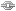### Information• Member: jt_x
• Title: depression
• Premiered: 2015-09-17
• Categories:
• Song:
• In Flames Come Clarity
• Anime:
• Comments: I edited this mostly in 2013 and recently found it so I finished it up in the past few days.
I made this video when I struggled with depression and wanted to portray how nebulous it feels and also how everything seems unreal and the struggle to find hope and to go on. enjoy.

programs used:

Sony Vegas 7.0e (man I'm old :0)
Avisynth plus r1551 (when I started)
Avisynth plus r1576 (somewhere in the middle)
Avisynth plus r1825
Baka Encoder v1.4.3
Ut Video v13.3 (when I started)
Ut Video v15.2

my post processing avisynth script:
### Import Video/Audio ###
AVISource("I:VideoEditing[jt_x]Blur.avi")
ConvertToYV12()
removegrainhd()

### Denoising ###
## KNLMeansCL() ##
#Spatial
#light: KNLMeansCL(D=0, A=2, h=1.2) #default
#medium: KNLMeansCL(D=0, A=4, h=3.6)
#strong: KNLMeansCL(D=0, A=6, h=6.0)
range(0,1178,"KNLMeansCL(D=0, A=6, h=6.0)")
range(1178,3012,"KNLMeansCL(D=0, A=4, h=3.6)")
range(3013,3045,"KNLMeansCL(D=0, A=6, h=6.0)")
range(3046,3093,"KNLMeansCL(D=0, A=4, h=3.6)")
range(3141,3209,"KNLMeansCL(D=0, A=4, h=3.6)")
range(3210,3213,"KNLMeansCL(D=0, A=4, h=3.6)")
range(3216,3231,"KNLMeansCL(D=0, A=2, h=1.2)")
range(3243,3246,"KNLMeansCL(D=0, A=4, h=3.6)")
range(3247,3285,"KNLMeansCL(D=0, A=4, h=3.6)")
range(3286,3311,"KNLMeansCL(D=0, A=2, h=1.2)")
range(3312,3453,"KNLMeansCL(D=0, A=6, h=6.0)")
range(3558,3649,"KNLMeansCL(D=0, A=4, h=3.6)")
range(3650,5527,"KNLMeansCL(D=0, A=4, h=3.6)")
range(5528,6385,"KNLMeansCL(D=0, A=6, h=6.0)")
### Denoising End ###

### Colorbending ###
flash3kyuu_deband()
### Colorbending End ###

### Sharpening / LineDarkening ###
vmtoon(sharpen=false,thinning=0,strength=35,luma_cap=203,threshold=8)
awarpsharp(10,2)
maa2(ss=4)
awarpsharp(10,2)
### Sharpening / LineDarkening End ###

### Levels ###
#Levels(input_low,gamma,input_high,output_low,output_high)
range(1579,1992,"Levels(20,1.00,255,0,270)")
range(2024,2050,"Levels(20,1.00,255,0,270)")
range(2096,2130,"Levels(20,1.00,255,0,270)")
tweak(sat=1.2)
### Levels End ###

Song Lyrics:
"Come Clarity"

Rushing through thirty
Getting older every day... by two
Drawing pictures of innocent times
Can you add color... inside these lines?

[Chorus x2:]
I want you to lead me
Take me somewhere
Don't want to live
In a dream... one more day

Sure it would change my perspective
I'm certain I would change today
I'm certain it would change our ways
Would things fall into place?

I want you to lead me
Take me somewhere
Just don't want to live
In a dream one more day [x2]

• Orig
• Visual
• Sound
• Synch
• Lip
• Effects
• Effort
• Re-View
• Overall
• 7.75
• 9.00
• 10.00
• 7.50
• 8.75
• 6.25
• 7.25